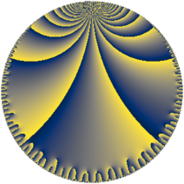# Properties

 Label 252.9.bhLevel $252$ Weight $9$ Character orbit 252.bh Rep. character $\chi_{252}(137,\cdot)$ Character field $\Q(\zeta_{6})$ Dimension $128$ Sturm bound $432$

# Related objects

## Defining parameters

 Level: $$N$$ $$=$$ $$252 = 2^{2} \cdot 3^{2} \cdot 7$$ Weight: $$k$$ $$=$$ $$9$$ Character orbit: $$[\chi]$$ $$=$$ 252.bh (of order $$6$$ and degree $$2$$) Character conductor: $$\operatorname{cond}(\chi)$$ $$=$$ $$63$$ Character field: $$\Q(\zeta_{6})$$ Sturm bound: $$432$$

## Dimensions

The following table gives the dimensions of various subspaces of $$M_{9}(252, [\chi])$$.

Total New Old
Modular forms 780 128 652
Cusp forms 756 128 628
Eisenstein series 24 0 24

## Trace form

 $$128 q + 923 q^{7} - 9374 q^{9} + O(q^{10})$$ $$128 q + 923 q^{7} - 9374 q^{9} + 17082 q^{11} - 1685 q^{13} + 106783 q^{15} - 31725 q^{17} + 92554 q^{19} - 560708 q^{21} - 1000449 q^{23} + 5000000 q^{25} + 1194525 q^{27} + 948816 q^{29} - 142784 q^{31} + 296468 q^{33} - 3488895 q^{35} + 273493 q^{37} - 107801 q^{39} + 2151648 q^{41} + 1131328 q^{43} - 11444734 q^{45} + 3561899 q^{49} - 10625137 q^{51} + 36078300 q^{53} + 13193184 q^{55} - 34145295 q^{57} + 34901410 q^{61} + 592101 q^{63} - 22149890 q^{67} - 104117929 q^{69} - 11872994 q^{73} - 80761361 q^{75} + 143491689 q^{77} + 107224174 q^{79} + 49223134 q^{81} + 28601250 q^{85} + 257943082 q^{87} - 122074911 q^{89} + 34062529 q^{91} - 217774979 q^{93} + 28782283 q^{97} - 141254777 q^{99} + O(q^{100})$$

## Decomposition of $$S_{9}^{\mathrm{new}}(252, [\chi])$$ into newform subspaces

The newforms in this space have not yet been added to the LMFDB.

## Decomposition of $$S_{9}^{\mathrm{old}}(252, [\chi])$$ into lower level spaces

$$S_{9}^{\mathrm{old}}(252, [\chi]) \cong$$ $$S_{9}^{\mathrm{new}}(63, [\chi])$$$$^{\oplus 3}$$$$\oplus$$$$S_{9}^{\mathrm{new}}(126, [\chi])$$$$^{\oplus 2}$$